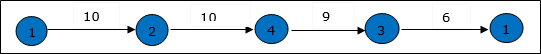# Travelling Salesman Model

Problem Statement

A traveler needs to visit all the cities from a list, where distances between all the cities are known and each city should be visited just once. What is the shortest possible route that he visits each city exactly once and returns to the origin city?

Solution

Travelling salesman problem is the most notorious computational problem. We can use brute-force approach to evaluate every possible tour and select the best one. For n number of vertices in a graph, there are (n – 1)! number of possibilities.

Instead of brute-force using dynamic programming approach, the solution can be obtained in lesser time, though there is no polynomial time algorithm.

Let us consider a graph G = (V, E), where V is a set of cities and E is a set of weighted edges. An edge e(u, v) represents that vertices u and v are connected. Distance between vertex u and v is d(u, v), which should be non-negative.

Suppose we have started at city 1 and after visiting some cities now we are in city j. Hence, this is a partial tour. We certainly need to know j, since this will determine which cities are most convenient to visit next. We also need to know all the cities visited so far, so that we don’t repeat any of them. Hence, this is an appropriate sub-problem.

For a subset of cities S Є {1, 2, 3, … , n} that includes 1, and j Є S, let C(S, j) be the length of the shortest path visiting each node in S exactly once, starting at 1 and ending at j.

When |S| > 1, we define C(S, 1) = ∝ since the path cannot start and end at 1.

Now, let express C(S, j) in terms of smaller sub-problems. We need to start at 1 and end at j. We should select the next city in such a way that

C(S,j)=minC(S−{j},i)+d(i,j)wherei∈Sandi≠jC(S,j)=minC(S−{j},i)+d(i,j)wherei∈Sandi≠j

Algorithm: Traveling-Salesman-Problem C ({1}, 1) = 0 for s = 2 to n do    for all subsets S Є {1, 2, 3, … , n} of size s and containing 1       C (S, 1) = ∞    for all j Є S and j ≠ 1       C (S, j) = min {C (S – {j}, i) + d(i, j) for i Є S and i ≠ j} Return minj C ({1, 2, 3, …, n}, j) + d(j, i)

Analysis

There are at the most 2n.n2n.n sub-problems and each one takes linear time to solve. Therefore, the total running time is O(2n.n2)O(2n.n2).

Example

In the following example, we will illustrate the steps to solve the travelling salesman problem.From the above graph, the following table is prepared.

 1 2 3 4 1 0 10 15 20 2 5 0 9 10 3 6 13 0 12 4 8 8 9 0

S = Φ

Cost(2,Φ,1)=d(2,1)=5Cost(2,Φ,1)=d(2,1)=5

Cost(3,Φ,1)=d(3,1)=6Cost(3,Φ,1)=d(3,1)=6

Cost(4,Φ,1)=d(4,1)=8Cost(4,Φ,1)=d(4,1)=8

S = 1

Cost(i,s)=min{Cost(j,s–(j))+d[i,j]}Cost(i,s)=min{Cost(j,s–(j))+d[i,j]}

Cost(2,{3},1)=d[2,3]+Cost(3,Φ,1)=9+6=15Cost(2,{3},1)=d[2,3]+Cost(3,Φ,1)=9+6=15

Cost(2,{4},1)=d[2,4]+Cost(4,Φ,1)=10+8=18Cost(2,{4},1)=d[2,4]+Cost(4,Φ,1)=10+8=18

Cost(3,{2},1)=d[3,2]+Cost(2,Φ,1)=13+5=18Cost(3,{2},1)=d[3,2]+Cost(2,Φ,1)=13+5=18

Cost(3,{4},1)=d[3,4]+Cost(4,Φ,1)=12+8=20Cost(3,{4},1)=d[3,4]+Cost(4,Φ,1)=12+8=20

Cost(4,{3},1)=d[4,3]+Cost(3,Φ,1)=9+6=15Cost(4,{3},1)=d[4,3]+Cost(3,Φ,1)=9+6=15

Cost(4,{2},1)=d[4,2]+Cost(2,Φ,1)=8+5=13Cost(4,{2},1)=d[4,2]+Cost(2,Φ,1)=8+5=13

S = 2

Cost(2,{3,4},1)={d[2,3]+Cost(3,{4},1)=9+20=29d[2,4]+Cost(4,{3},1)=10+15=25=25Cost(2,{3,4},1)={d[2,3]+Cost(3,{4},1)=9+20=29d[2,4]+Cost(4,{3},1)=10+15=25=25

Cost(3,{2,4},1)={d[3,2]+Cost(2,{4},1)=13+18=31d[3,4]+Cost(4,{2},1)=12+13=25=25Cost(3,{2,4},1)={d[3,2]+Cost(2,{4},1)=13+18=31d[3,4]+Cost(4,{2},1)=12+13=25=25

Cost(4,{2,3},1)={d[4,2]+Cost(2,{3},1)=8+15=23d[4,3]+Cost(3,{2},1)=9+18=27=23Cost(4,{2,3},1)={d[4,2]+Cost(2,{3},1)=8+15=23d[4,3]+Cost(3,{2},1)=9+18=27=23

S = 3

Cost(1,{2,3,4},1)=⎧⎩⎨d[1,2]+Cost(2,{3,4},1)=10+25=35d[1,3]+Cost(3,{2,4},1)=15+25=40d[1,4]+Cost(4,{2,3},1)=20+23=43=35Cost(1,{2,3,4},1)={d[1,2]+Cost(2,{3,4},1)=10+25=35d[1,3]+Cost(3,{2,4},1)=15+25=40d[1,4]+Cost(4,{2,3},1)=20+23=43=35

The minimum cost path is 35.

Start from cost {1, {2, 3, 4}, 1}, we get the minimum value for d [1, 2]. When s = 3, select the path from 1 to 2 (cost is 10) then go backwards. When s = 2, we get the minimum value for d [4, 2]. Select the path from 2 to 4 (cost is 10) then go backwards.

When s = 1, we get the minimum value for d [4, 2] but 2 and 4 is already selected. Therefore, we select d [4, 3] (two possible values are 15 for d [2, 3] and d [4, 3], but our last node of the path is 4). Select path 4 to 3 (cost is 9), then go to s = Φ step. We get the minimum value for d [3, 1] (cost is 6).## One thought on “Travelling Salesman Model”

error: Content is protected !!